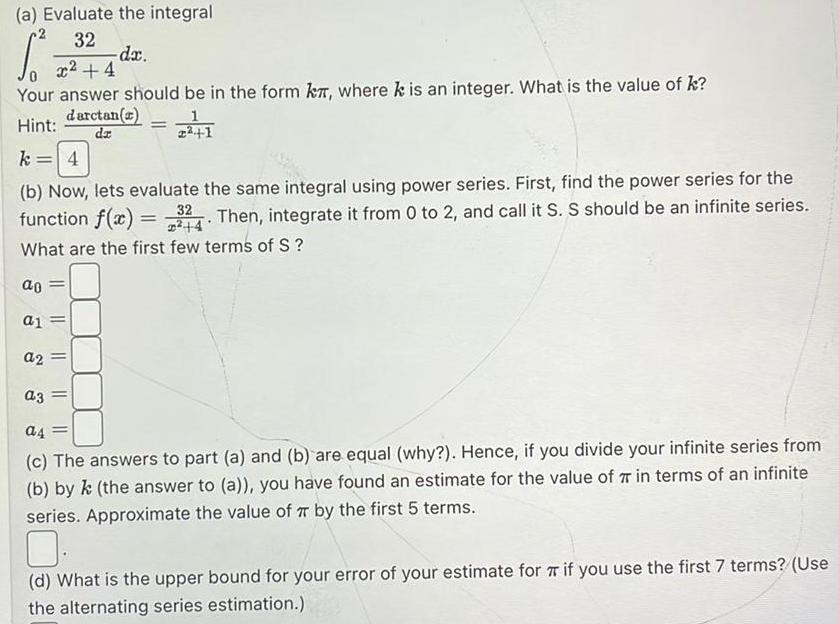Question:

# a Evaluate the integral 32 S x 4 Your answer should be in

Last updated: 9/19/2023a Evaluate the integral 32 S x 4 Your answer should be in the form k where k is an integer What is the value of k darctan a Hint dz dx a1 a2 a3 1 224 1 k 4 b Now lets evaluate the same integral using power series First find the power series for the function f x 324 Then integrate it from 0 to 2 and call it S S should be an infinite series 4 What are the first few terms of S ao a4 c The answers to part a and b are equal why Hence if you divide your infinite series from b by k the answer to a you have found an estimate for the value of 7 in terms of an infinite series Approximate the value of TT by the first 5 terms d What is the upper bound for your error of your estimate for if you use the first 7 terms Use the alternating series estimation